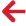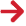﻿ PipeLay > Technical Notes > Technical Note 7 - Calculation of Moment/Curvature Curves for Pipe Elements with a Stress/Strain Material Specification > Calculation with External Coatings and Tension > Calculation of Slippage in the Outermost Coating

# Calculation of Slippage in the Outermost CoatingIf a BBR value is specified for the outermost coating, then slippage of this layer can occur. As mentioned already, the slippage is quantified by means of a slippage coefficient. The computation of a suitable slip coefficient also requires an iterative solution, as outlined over the following steps:

ii.Steps 2a to 2b from the existing moment calculation algorithm are executed, but the curvature of the outermost layer is assumed to be the product of the global curvature times the slip coefficient. As per the without slippage case, each layer in the slice is to have its own stress values.

iii.For each slice the stress in the outermost layer is compared to the BBR user input.

a.If the all the stress values are less or equal than the BBR, then the slip coefficient is determined.

b.Otherwise, the slip coefficient is decremented by a small value and steps ii to iii are repeated.

iv.Steps ii to iii are repeated in a similar fashion for the compressive region of the pipe. The accepted slip coefficient is the minimum of the two coefficients from both regions.

The iteration on slip coefficient occurs within the iteration loop on the neutral axis position and so only when both iteration processes have converged is the bending moment for a particular curvature determined successfully.